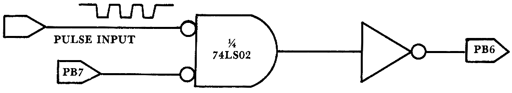Back to the index of Compute II

COMPUTE II ISSUE 1 / APRIL/MAY 1980 / PAGE 36

Improved Pulse Counting Software For The 6522 VIA

Marvin L. De Jong
Dept. of Mathematics-Physics
The School of the Ozarks
Pt. Lookout, MO 65726

Ever since I began playing with the 6522 I have been trying to find a program that would use the 6522 to count pulses for an exact one second interval. By exact I mean one million clock cycles, not one million plus or minus several instruction intervals. Of course, it should be noted that if the system clock frequency is not exactly one Megahertz then an error of several instruction intervals may not be particularly important. In this connection, the measurements I have made of clock frequencies on a few KIM-1s and one AIM 65 show that errors of several hundred parts per million are not unusual, so if your twenty-four hour clock runs slow or fast, do not be surprised.

In any case, assuming that the system clock frequency is precise to say one part per million, the program supplied in this note will count pulses for an interval that is as precise as the system clock frequency. The assembly language program to count pulses for exactly one second (one million clock cycles) is given in Table 1, and the simple interface circuit it requires is given in Figure 1. A BASIC program to convert the pulse count to decimal and display it is given in Table 2. This program works on my AIM 65, and it will probably have to be modified for other machines.

The assembly language program in Table 1 makes use of the T1 timer in its one-shot mode with PB7 enabled. That is, the T1 timer is programmed to produce a time interval of 50,000 clock cycles, and during that interval of 0.05 s PB7 is held at logic zero. Refer to Figure 1 and note that when PB7 is at logic zero the pulses from some external device will be gated to PB6, the pulse-counting pin for the T2 counter/timer. In order to produce pulse-counting intervals that are longer than 0.05 s, the T1 timer is reloaded and started N times, where N is an eight-bit number stored in a memory location labeled CNTR in Table 1. Thus, if N = 2 the counting interval is 0.1 s, if N = 20 the counting interval is 1.0 s, and if N = 200 the counting interval is 10 s. These numbers must be converted to hexadecimal numbers before using them in the program.

While T1 is timing-out it is read continuously so that it may be reloaded and started after exactly 50,000 clock cycles. This prevents PB7 from reaching logic one any time during the N timing intervals. If we were to allow T1 to time-out and then reload and start it, PB7 would toggle from logic zero to logic one and back to logic zero, with the possibility of producing an extraneous count on PB6. Thus, the program loop starting from REPEAT in Table 1 and ending with DUMMY in the same listing is tuned to take exactly 50,000 clock cycles. Each time through the loop N is decremented, until it reaches zero at which time T1 is finally allowed to time-out for the last time.

When T1 times out for the last time, no more pulses will reach PB6. At this time the interrupt flag register (IFR) on the 6522 is read first. If the T2 flag is set, then the pulse count was greater than \$010000 (6553610) because the T2 counter was initially loaded with \$FFFF. If the T2 interrupt flag (IFR5) is set, then the most-significant byte, PLUSHI, of the pulse-count storage locations is incremented. Otherwise it is cleared. After this operation, the T2 counter is read and the resulting pulse counts are loaded into PLSMI, the middle byte of the three-byte pulse-count storage locations, and PLSLO, the least-significant byte of the pulse-count storage locations. The program then uses a JMP instruction to return to the BASIC calling program given in Table 2. Other BASICs may use a different return technique.

The most obvious application of pulse counting is a simple frequency meter. The programs and interface described here will count at a maximum pulse count of 131,071 counts during whatever counting interval (0.1 s, 1.0 s, or 10 s) you choose. Note that 131,071 = \$01FFFF. Other applications include voltage-to-frequency converters and temperature-to-frequency converters. Commercial tachometer pickups produce a pulse rate that is proportional to the angular velocity (RPM) of a rotating shaft. The 6522 can be used to measure this pulse rate and the microcomputer can convert it to rotations per minute. The 6522 can also be interfaced to Geiger counters (GM tubes) or scintillation detectors to count nuclear events. There are a variety of new transducers appearing (temperature, light intensity, pressure) that can be used with a V/F converter to produce a pulse rate that is directly proportional to the physical quantity being measured. Although direct analog-to-digital (A/D) conversion is faster than pulse counting, it usually requires a much more sophisticated interface. In applications where speed is not a problem, investigate the possibility of using this simple program and interface.

Table 1. Simple pulse counting program for the 6522.

Table 2. Counting Pulses with a BASIC program.

```10 REM THIS PROGRAM REQUIRES THE MACHINE LANGUAGE ROUTINE IN TABLE 1.
20 POKE 04,00:POKE 05,15
30 Y=USR(0)
40 X=PEEK(49)+256*PEEK(50)+65536*PEEK(51)
50 PRINT X; "PULSES PER SECOND"
60 GOTO 30
70 END
```

Figure 1.Interface circuit for the pulse-counting program of Table 1. The inverter can be implemented with one of the other gates on the 74LS02 chip. The incoming pulse train must be at TTL logic levels.